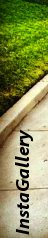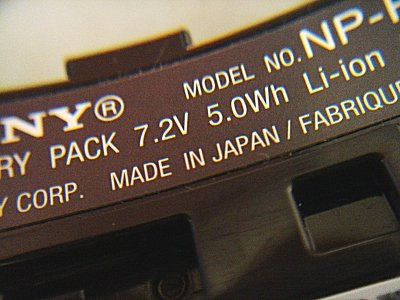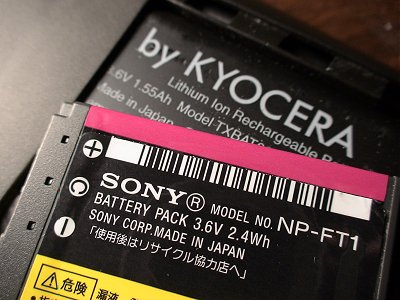Definitions

## Battery Capacity Conversion

Sony has the tendency to specify lithium ion battery capacity in watt-hour (Wh) instead of the more popular milliamp-hour (mAh) that everyone else uses. This deviation makes it hard to compare battery capacity between a battery specified in Wh and one specified in mAh. Perhaps Sony did this on purpose so that consumers with no electrical background can only comparatively shop among Sony battery packs.Good news is that the conversion between watt and amp is fairly straight forward. The relationship between watt, amp, and volt is shown in the mathematical equation below.

```              watt = amp * volt
```

Using the equation above and knowing two of the three variables, you can calculate the third variable. When you compare between two battery packs for your digital camera or your digital camcorder, the voltage is the same and known. Therefore it is a matter of plugging in the capacity specification in Wh or mAh to obtain the capacity in the other unit.

### ExampleSony infoLITHIUM NP-FT1 battery pack that came with the DSC-T11 is a 3.6V 2.4Wh battery. On the other hand, the TXBAT091 Lithium Ion Rechargeable Battery that came with the Kyocera QCP-6035 Palm-Powered SmartPhone is a 3.6V 1.55Ah battery. Which battery has a larger capacity?

```              Wh = 1.55Ah * 3.6V
Wh = 5.58
```

Therefore the TXBAT091 has a capacity that is more than twice as large as the NP-FT1. You can also convert Wh to Ah by rebalancing the early equation to the one shown below.

```              amp = watt / volt
```

Thus, for the NP-FT1 battery:

```              Ah = 2.4Wh / 3.6V
Ah = 0.66
```

### Amp to Milliamp

So far, we have been converting between Ah and Wh. But most battery packs are specified in mAh, which is simply milliamp-hour. To convert amp to milliamp, all you have to do is multiply amp by one thousand. To convert milliamp to amp, simply divide milliamp by one thousand.

For the NP-FT1 battery pack:

```              mAh = 0.66Ah * 1000
mAh = 666
```

### Why is Battery Capacity Specified in Ampere-Hour?

An ampere is a unit for measurement for the amount of electricity current flowing through a circuit. One ampere is the same as one coulomb of electric charge flowing past any point per second. It is also the same as current produced by one volt of electricity applied across a 1-ohm resistance.

One ampere-hour is equal to a current of one ampere flowing for one hour. So, if you have a two ampere-hour battery, then it has the capacity to flow a two-ampere current for one hour. Or you can use the same battery to flow a one-ampere current for two hours. Therefore, a larger Ah always specifies higher capacity.